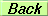# SEMINAR

SU(5)$otimes$Z$_{13}$ Grand Unification Model

Edison T. Franco

(CFTP-IST & IFT-UNESP, S.Paulo, Brazil)

Sala P7, IST, Edifício Matemática
Thursday, February 5th, 2009 at 04:00 PM

Abstract

We propose an $SU(5)$ grand unified model with an invisible axion and the unification of the three coupling constants which is in agreement with the values, at $M_Z$, of $\alpha$, $\alpha_s$, and $\sin^2\theta_W$. A discrete, anomalous, $Z_{13}$ symmetry implies that the PQ symmetry is an automatic symmetry of the classical Lagrangian protecting, at the same time, the invisible axion against possible semi-classical gravity effects. Although the unification scale is of the order of the PQ scale the proton is stabilized by the fact that in this model the standard model fields form the $SU(5)$ multiplets completed by new exotic fields and, also, because it is protected by the $Z_{13}$ symmetry.

© CFTP 2019